A Good Choice of Ridge Parameter with Minimum Mean Squared Error | OMICS International
Journal of Biometrics & Biostatistics

Like us on:

# A Good Choice of Ridge Parameter with Minimum Mean Squared Error

Iguernane M*

Department of Mathematics, Faculty of Science, King Khalid University, Saudi Arabia

*Corresponding Author:
Iguernane M
Department of Mathematics
Faculty of Science
King Khalid University
Saudi Arabia
Tel: + 00966556300495
E-mail: [email protected]

Received Date: March 03, 2016; Accepted Date: March 21, 2016; Published Date: March 28, 2016

Citation: Iguernane M (2016) A Good Choice of Ridge Parameter with Minimum Mean Squared Error. J Biom Biostat 7: 289. doi:10.4172/2155-6180.1000289

Copyright: © 2016 Iguernane M. This is an open-access article distributed under the terms of the Creative Commons Attribution License, which permits unrestricted use, distribution, and reproduction in any medium, provided the original author and source are credited.

Visit for more related articles at Journal of Biometrics & Biostatistics

#### Abstract

In this paper, the problem of estimating the regression parameters is considered in a multiple regression model Y= X α + u hen the multicollinearity is present. Two suggested methods of finding the ridge regression parameter k are investigated and evaluated in terms of Mean Square Error (MSE) by simulation techniques. A number of factors that may affect the properties of these methods have been varied. Results of a simulation study indicate that with respect to MSE criteria, the suggested estimators perform better than both the ordinary least squares (OLS) estimator and the other estimators discussed here.

#### Keywords

Multicollinearity; Ridge Regression; Simulation

#### Introduction

In multiple regressions it is known that the parameter estimates, based on minimum residual sum of squares, have a high probability of being unsatisfactory if the prediction vectors, X, and are multicollinear.

In fact, the question of multicollinearity is not of existence, but of degree. In the situation when the prediction vectors are far from being orthogonal, i.e. when strong multicollinearities exist in X, Horel and Kennard  suggested ridge regression to deal with the problem of estimating the ridge regression parameters. However, if the degree of multicollinearity in X is not strong, then the data are near-orthogonal. In this situation, various estimators (called shrunken estimators, introduced by Stein  are known to dominate the OLS estimator, as is shown in many simulation studies comparing shrunken estimators among themselves and with the OLS estimator, Vinod  and Gunst and Mason .

To discuss the multicollinearity problem, let us consider the standard multiple linear regression model;

Y= X α + u,          (1)

Where (Y= T × 1) consists of the observations on the dependent variable, (Y= T × p) is the matrix of observations on the explanatory variables, (Y= T × 1) is the residual vector. Obviously, we have;

Where (Y= T × 1) consists of the observations on the dependent variable, (Y= T × p) is the matrix of observations on the explanatory variables, (Y= T × 1) is the residual vector. Obviously, we have;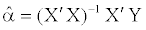(2)

Where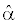is the OLS estimator for α . If we denote X′X by Q and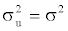, then the variance ofis given by;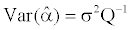(3)

Denoting the eigenvalue of Q by λi , it can be shown that;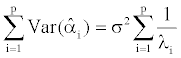(4)

In case of severe multicollinearity, Q becomes almost singular, which means that one or more of the eigenvalues λi are close to zero. The effect on the OLS estimator is obvious from Eq. (4). The variance of the estimator becomes large and the estimates are strongly correlated. A further effect of multicollinearity is that the parameter estimates tends to become "too large", which is shown by the fact that;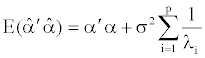(5)

The measurement of the severity of the multicollinearity in no straight-forward matrix, except in the case when the number of explanatory variables is just two. In this case, the simple correlation coefficient between the explanatory variables contains all the relevant information. When the number of explanatory variables is large than two, the pairwise correlation coefficients are still important. However, it is possible for the multicollinearity to be quite severe, even if all simple correlation coefficients are only moderately large. The fundamental reason for all the trouble is the fact that the matrix Q is almost singular. Thus, the value of the determinant of this matrix is an indicator of the severity of the problem.

In an effort to circumvent the problems caused by multicollinearity, Horel and Kennard  proposed a biased estimator usually called ridge regression and defined as follows;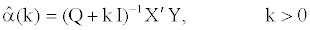(6)

It is almost possible to find a value of k for which;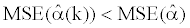(7)

This result is obtained by Horel and Kennard . This means that it is always possible to find a value of k that leads to a smaller MSE than in the case of OLS estimator. There are many different techniques for estimating the ridge parameter k have been proposed (see for example, Horel, Kennard and Baldwin , Gibbons , Kibria , Khalaf and Shukur , Khalaf , Khalaf , Khalaf and Iguernane .

The plan of this paper is as follows: In Section 2, the two proposed methods to estimate the ridge regression parameter k are described. Then we illustrate the simulation in Section 3, after that the results of simulation are given in Section 4. And finally, a summary and conclusion are presented in Section 5.

#### The Proposed Estimators

Horel and Kennard proved that the value ki which minimize the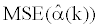, given by;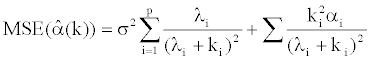(8)

is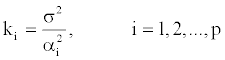(9)

Where σ2 represents the error variance of model (1), i α is the ith element of α . However, the optimal value of ki fully depends on the unknown σ2 and i α and they must be estimated from the observed data. That is why Horel and Kennard  suggested to replace σ2 and αi by their corresponding unbiased estimators. That is;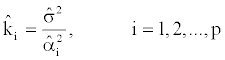(10)

Where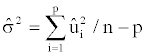is the unbiased estimator of σ2 and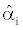is the ith element ofwhich is unbiased estimator of α . For this estimator, we use the acronym HK.

Based on Eq. (10), we will review some methods as follows;

(1) Horel et al.  proposed a different estimator of k by taking the harmonic mean of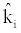in Eq. (10). That is;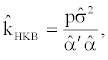(11)

Whereis the OLS estimator of α . For this estimator, we use the acronym HKB.

(2) From the Bayesian point of view, Lawless and Wang  suggested an estimator of k. The corresponding estimator of HKB is given by;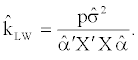(12)

For this estimator, we use the acronym LW.

Now, the modifications we suggest are accomplished by multiplying the amount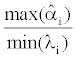to the above estimators. Therefore, the two new proposed methods for estimating the ridge parameter k are as follows;

New estimator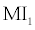; by using Eq. (11) which produce the following estimator;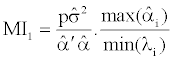(13)

New estimator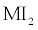, by using Eq. (12) which gives the following estimator;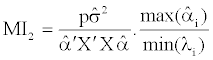(14)

#### The Simulation

In this section, we present a simulation to illustrate the performance of the ridge regression estimator based on the suggested estimators when compared with the OLS estimator and the ridge regression estimators, based on HK, HKB and LW. The properties of these estimators will be compared in terms of MSE criterion. To compare between these five methods, we prefer that who give the smallest MSE.

Following Mu1 niz and Kibria , the explanatory is generated by;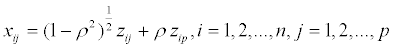(15)

Where zij are generated using the standard normal distribution and the dependent variable is then determined by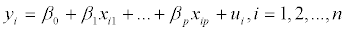(16)

Where n is the number of observations, ui are i.i.d. pseudo-random numbers, and β0 is taken to be zero. We choose the parameter values so that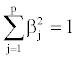which is a common restriction in simulation studies.

Three factors can affect these properties; the first factor is that of the sample size (n), the second one is the degree of correlation between the explanatory variables, and, finally, the error variance as a third factor. In other words, we will study the consequences of varying n, degree of correlation and error variance.

To investigate the effect of sample sizes on the properties of all estimators under consideration, we used samples of the size; 10, 20, 70 and 150 which may cover situations of small and large samples.

Two models are used, one is the 6-factor structure, and another is the 8-factor structure. Since our primary interest lies on investigating the properties of our proposed approaches to minimize the MSE, thus the different degrees of correlation between the variables included in the two models, has been used. We choose these values equal to 0.6, 0.8, 0.94 and 0.99. These values will cover a wide range of moderate and strong correlation among explanatory variables. The values of σ2 are considered which are 0.2, 0.6 and 1.

To investigate the performance of the different proposed ridge regression estimators and the OLS, we calculate the MSE using the following equation;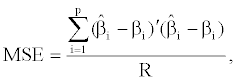(17)

Where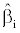is the estimator of β obtained from the OLS or the other different ridge parameters and R equals 9000 which corresponding to the number of replications used in the simulation.

#### The Results

In this section we present the results of our simulation concerning the properties of our suggested estimators and that for the others for choosing the ridge regression parameter k, when multicollinearity among the columns of the design matrix exists.

It is known that goodness and accuracy of an estimator is quantified through the MSE criterion. We now compare the MSE among the different methods used to develop the ridge regression parameter k. Small MSE indicates a good performance of the respective suggested method. In what follows, we go through Tables 1 and 2.

σ2 = 0.2

ρ n OLS HK HKB LW MI1 MI3
10 82.02 29.46 20.63 5.29 4.88 5.51
0.6 20 18.83 8.98 6.24 5.25 3.11 3.02
70 3.90 2.97 2.04 3.35 1.19 2.55
150 1.694 1.490 1.141 1.640 1.032 1.621

σ2 = 0.6

ρ n OLS HK HKB LW MI1 MI3
10 6.62 3.42 2.34 4.36 1.37 2.05
0.6 20 1.511 1.281 0.972 1.467 0.856 1.432
70 0.3151 0.3067 0.2838 0.3147 0.3049 0.3150
150 0.139508 0.137920 0.133043 0.139475 0.138628 0.139504

σ2 = 1

ρ n OLS HK HKB LW MI1 MI3
10 3.180 1.955 1.405 2.696 0.954 1.947
0.6 20 0.7502 0.6861 0.5708 0.7447 0.5921 0.7449
70 0.156666 0.154544 0.148265 0.156620 0.155305 0.156660
150 0.0673158 0.0669337 0.0657255 0.0673120 0.0672098 0.0673156

σ2 = 0.2

ρ n OLS HK HKB LW MI1 MI3
10 158.36 50.68 36.84 5.29 4.82 5.62
0.8 20 36.70 15.34 10.51 6.65 3.13 3.32
70 7.45 4.74 2.97 5.88 0.83 2.38
150 3.30 2.58 1.69 3.13 0.82 2.79

σ2 = 0.6

ρ n OLS HK HKB LW MI1 MI3
10 17.52 7.29 4.83 8.17 1.51 1.69
0.8 20 3.946 2.743 1.765 3.633 0.760 2.796
70 0.8338 0.7736 0.6250 0.8308 0.6485 0.8312
150 0.36411 0.35237 0.31523 0.36386 0.34354 0.36402

σ2 = 1

ρ n OLS HK HKB LW MI1 MI3
10 6.322 3.264 2.112 4.994 0.798 2.590
0.8 20 1.458 1.234 0.890 1.441 0.735 1.422
70 0.29872 0.29058 0.26414 0.29858 0.28630 0.29868
150 0.132156 0.130628 0.125055 0.132144 0.131098 0.132154

σ2 = 0.2

ρ n OLS HK HKB LW MI1 MI3
10 544.70 174.57 120.38 4.55 5.11 5.89
0.94 20 127.49 45.91 31.86 6.79 3.81 4.87
70 26.29 11.60 7.61 13.54 0.94 0.52
150 11.63 6.23 3.92 9.73 0.35 2.85

σ2 = 0.6

ρ n OLS HK HKB LW MI1 MI3
10 63.54 21.17 15.03 16.40 1.81 1.27
0.94 20 14.42 6.94 4.49 11.28 0.44 2.62
70 2.974 2.287 1.461 2.936 0.618 2.833
150 1.276 1.126 0.814 1.273 0.686 1.271

σ2 = 1

ρ n OLS HK HKB LW MI1 MI3
10 21.86 8.55 5.74 13.70 0.66 2.32
0.94 20 5.167 3.281 2.005 4.967 0.464 3.960
70 1.054 0.946 0.703 1.052 0.645 1.052
150 0.4675 0.4460 0.3767 0.4674 0.4111 0.4675

σ2 = 0.2

ρ n OLS HK HKB LW MI1 MI3
10 3712 1132 782 2.46 5.49 5.99
0.99 20 832 288 194 2.97 4.80 5.88
70 177 65 44 21 2.23 2.56
150 78 29 20 32 0.74 0.20

σ2 = 0.6

ρ n OLS HK HKB LW MI1 MI3
10 424 129 91 27 2.80 3.42
0.99 20 94 33 22 34 0.88 0.32
70 19.27 8.68 5.56 17.58 0.10 4.97
150 8.59 4.88 2.97 8.44 0.18 6.82

σ2 = 1

ρ n OLS HK HKB LW MI1 MI3
10 143 45 32 40 1.15 0.67
0.99 20 34 13 9 27 0.16 2.32
70 7.085 4.219 2.544 6.996 0.236 6.174
150 3.1065 2.3059 1.4289 3.0989 0.4758 3.0726

Table 1: Estimated MSE when p = 6.

σ2 = 0.2

ρ n OLS HK HKB LW MI1 MI3
10 315 89 52 8.93 7.09 7.66
0.6 20 30 14 8.92 7.57 4.49 4.37
70 5.57 4.40 2.78 4.83 1.42 3.31
150 2.40 2.15 1.57 2.33 1.25 2.26

σ2 = 0.6

ρ n OLS HK HKB LW MI1 MI3
10 40 12 7.86 9.23 3.48 2.71
0.6 20 3.37 2.689 1.78 3.15 1.15 2.685
70 0.61899 0.600 0.531 0.617 0.566 0.61831
150 0.268472 0.265264 0.251895 0.268371 0.264139 0.268450

σ2 = 1

ρ n OLS HK HKB LW MI1 MI3
10 13.59 5.05 3.23 6.60 1.87 2.50
0.6 20 1.2299 1.115 0.867 1.218 0.812 1.2102
70 0.22178 0.21931 0.20908 0.22172 0.21881 0.22176
150 0.094576 0.094157 0.092320 0.094571 0.094359 0.094575

σ2 = 0.2

ρ n OLS HK HKB LW MI1 MI3
10 658 165 111 11.41 6.90 7.71
0.8 20 59 25 15 10 4.50 4.74
70 10.68 7.12 4.04 8.57 0.99 2.87
150 4.677 3.783 2.327 4.458 0.869 3.785

σ2 = 0.6

ρ n OLS HK HKB LW MI1 MI3
10 68.49 20.91 12.41 14.66 3.26 2.49
0.8 20 6.66 4.56 2.63 6.04 0.84 3.85
70 1.198 1.121 0.868 1.194 0.819 1.193
150 0.5215 0.5077 0.4467 0.5212 0.4768 0.5214

σ2 = 1

ρ n OLS HK HKB LW MI1 MI3
10 28.39 8.88 5.12 11.50 1.64 2.73
0.8 20 2.369 1.982 1.290 2.336 0.827 2.248
70 0.4292 0.4190 0.3737 0.4290 0.4000 0.4291
150 0.18700 0.18512 0.17561 0.18698 0.18449 0.18699

σ2 = 0.2

ρ n OLS HK HKB LW MI1 MI3
10 2289 637 369 12.55 7.16 7.90
0.94 20 209 80 47 11.32 5.39 6.70
70 37 17 10.33 20 1.30 0.55
150 16.54 9.65 5.34 14.05 0.40 3.49

σ2 = 0.6

ρ n OLS HK HKB LW MI1 MI3
10 235 61 37 28 3.67 2.97
0.94 20 23.39 11.42 6.41 17.75 0.58 2.70
70 4.24 3.35 1.96 4.19 0.57 3.96
150 1.8343 1.6412 1.1203 1.8301 0.7647 1.8244

σ2 = 1

ρ n OLS HK HKB LW MI1 MI3
10 90 25 15 27 1.80 2.16
0.94 20 8.31 5.22 2.85 7.94 0.41 5.45
70 1.525 1.382 0.967 1.523 0.739 1.5208
150 0.660364 0.633101 0.516851 0.660168 0.534142 0.660196

σ2 = 0.2

ρ n OLS HK HKB LW MI1 MI3
10 14558 4061 2407 14.92 7.46 7.99
0.99 20 1398 516 299 6.03 6.65 7.88
70 253 100 58 37 3.14 3.31
150 110 44 27 50 1.03 0.21

σ2= 0.6

ρ n OLS HK HKB LW MI1 MI3
10 1870 497 407 58 4.83 5.85
0.99 20 156 58 34 55 1.36 0.47
70 27 13 7.58 25 0.09 6.09
150 12.17 7.28 3.97 11.965 0.13 8.96

σ2 = 1

ρ n OLS HK HKB LW MI1 MI3
10 588 160 98 72 2.86 2.01
0.99 20 56 23 13 43 0.23 2.35
70 10.18 6.29 3.40 10.06 0.17 8.40
150 4.505 3.432 1.967 4.495 0.426 4.433

Table 2: Estimated MSE when p = 8.

It is noted that our suggested estimators MI1 and MI2 produce a small MSE among all the parameters under consideration in both models, in particular MI1, when the sample size and the correlation coefficient are large. We also noted that the HKB estimator performs well comparative with the OLS estimator and the other ridge estimator.

In Model (2), it is clear that MI1 is better than all other estimators, especially, when n is large followed by HKB.

#### Discussions and Conclusions

In this paper, we studied the properties of two modifications of Horel et al. , given by (11), and Lawless and Wang , defined by (12), proposed approaches for choosing the ridge parameter k when the multicollinearity among the explanatory variables exists.

The investigating has been done using simulation technique where, in addition to the different multicollinearity levels, the numbers of observations and the error variance have been varied. For each combination, we have used good replication. The evaluation of our suggested methods, given by Eqs (13) and (14), has been done by comparing the MSEs between these methods and those of HK, HKB and LW. We found that the performance of our suggested methods, in particular, outperform the others in almost all cases, especially when the variance of the residual and the sample size are large. The results also indicate that all methods produced a smaller MSE than that of the OLS, and the OLS estimator gets the worst in all cases with regard to the MSE criterion.

#### References

Select your language of interest to view the total content in your interested language

### Article Usage

• Total views: 9439
• [From(publication date):
April-2016 - Dec 10, 2019]
• Breakdown by view type
• HTML page views : 9273Can't read the image? click here to refresh